Next: Plate, steady 1-D Helmholtz. Up: Rectangular Coordinates. Helmholtz Equation. Previous: Infinite body,rectangular coordinate, steady

### Semi infinite body, steady 1-D Helmholtz.

X10 Semi-infinite body, 0 < x <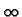, with G = 0 (Dirichlet) at x = 0.

 GX10(x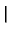x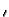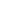) =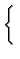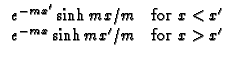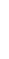X20 Semi-infinite body, 0 < x <, with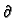G/x = 0 at x=0.

 GX20(xx) =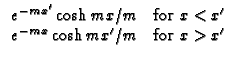X30 Semi-infinite body, 0 < x <, with - kG/x + hG = 0 (convection) at x = 0. Note that B = h/(km).

 GX30(xx) =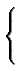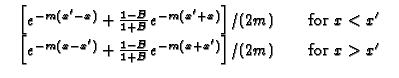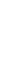Kevin D. Cole
2002-12-31